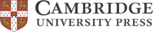## Well-posed and ill-posed behaviour of the -rheology for granular flow

T. Barker, D. G. Schaeffer, P. Bohorquez, J. M. N. T. Gray
• Journal of Fluid Mechanics, August 2015, Cambridge University Press
• DOI: 10.1017/jfm.2015.412

# Well-posed and ill-posed behaviour of the μ(I)-rheology for granular flow

In light of the successes of the Navier–Stokes equations in the study of fluid flows, similar continuum treatment of granular materials is a long-standing ambition. This is due to their wide-ranging applications in the pharmaceutical and engineering industries as well as to geophysical phenomena such as avalanches and landslides. Historically this has been attempted through modification of the dissipation terms in the momentum balance equations, effectively introducing pressure and strain-rate dependence into the viscosity. Originally, a popular model for this granular viscosity, the Coulomb rheology, proposed rate-independent plastic behaviour scaled by a constant friction coefficient . Unfortunately, the resultant equations are always ill-posed. Mathematically ill-posed problems suffer from unbounded growth of short-wavelength perturbations, which necessarily leads to grid-dependent numerical results that do not converge as the spatial resolution is enhanced. This is unrealistic as all physical systems are subject to noise and do not blow up catastrophically. It is therefore vital to seek well-posed equations to make realistic predictions. The recent μ(I)-rheology is a major step forward, which allows granular flows in chutes and shear cells to be predicted. This is achieved by introducing a dependence on the non-dimensional inertial number I in the friction coefficient μ. In this paper it is shown that the μ(I)-rheology is well-posed for intermediate values of I, but that it is ill-posed for both high and low inertial numbers. This result is not obvious from casual inspection of the equations, and suggests that additional physics, such as enduring force chains and binary collisions, becomes important in these limits. The theoretical results are validated numerically using two implicit schemes for non-Newtonian flows. In particular, it is shown explicitly that at a given resolution a standard numerical scheme used to compute steady-uniform Bagnold flow is stable in the well-posed region of parameter space, but is unstable to small perturbations, which grow exponentially quickly, in the ill-posed domain.

### Why is it important?

The μ(I)-rheology represents a major breakthrough in the study of liquid-like flows of granular material. We find that for many commonly studied flows the model is well-posed but when the inertial number I is too small or too large, the equations are ill-posed. These findings mean that numerical simulations of flows that include these regimes will give erroneous and often catastrophic results. As ill-posedness is defined as an unbounded growth of infinitesimal wavelength perturbations, making the solution grid in numerical calculations finer actually speeds up the growth of noise. This growth leads to unphysical results with initially small imperfections coming to dominate the solution and ever larger magnitudes at ever faster rates are observed as the grid-scale is reduced. We prove these claims by means of an asymptotic linear stability analysis performed especially for small wavelength perturbations. We then solve the full two-dimensional transient equations as a validation of our mathematical predictions. An important test case comes from flows on inclined frictional planes. The μ(I)-rheology was designed to capture the observation that such flows were only steady and uniform for a finite range of non-zero inclination angles. Application of our criteria for well-posedness, and related numerical simulations, confirm that for a large part of this range the flows are indeed steady and uniform. However, we show that for larger angles, still within the observed steady range, numerical computations spontaneously generate perturbations that grow, dominate the solutions and ultimately cause numerical methods to fail.

### Perspectives

##### Thomas Barker
University of Manchester

This work and publication has kick-started a journey of scientific and mathematical exploration that highlights some of the biggest struggles that the granular flow community currently faces. Low inertial numbers correspond to material that is close to static and where many experimental observations call into question our key hypotheses. Very large interial numbers can only be possible when the grains are behaving more like a gas than a fluid or solid, another concern for mean-field continuum models. Future work can now be directed at precisely these two limits in order to produce satisfactory mathematical models and the resulting tools that can be employed in real-world scenarios. The study of any physical system should take care of the precise mathematical formulation used to describe it. In addition users of these models should be aware of the limitations inherent in the description. Whilst being mainly a mathematical and numerical study, our results cast doubt on the physical validity of the μ(I)-rheology in the cases that the resultant equations are ill-posed. This result may be seen as a positive step on the road to a full and satisfactory description of granular materials. Since publication, two strategies have been employed that ensure that dynamic equations for granular flow are well-posed. The first is to include additional, physically motivated, terms and equations that both improve the matching with experimental data and avoid the numerical and philosophical issues surrounding ill-posedness. One conclusive and simple addition is to allow the local solids volume fraction φ to vary. This leads to compressible μ(I)-equations which can be made well-posed given certain criteria on the dissipative terms (Barker, Schaeffer, Shearer & Gray Proc. R. Soc. A 2017 and Heyman, Delannay, Tabuteau & Valance J. Fluid Mech. 2017). It has also been shown that well-posed incompressible μ(I)-equations are possible if the functional form of the μ(I)-curve is changed in the previously ill-posed ranges (Barker & Gray J. Fluid Mech. 2017). This allows for accurate, reliable simulations for all inertial numbers below a very large maximal value.

http://dx.doi.org/10.1017/jfm.2015.412

The following have contributed to this page: Professor John M N T Gray and Thomas BarkerIn partnership with: